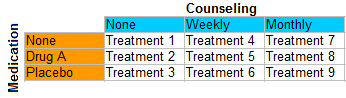# Statistical Treatment

Statistics Definitions > Statistical Treatment

## What is Statistical Treatment?

Statistical treatment can mean a few different things:

1. In Data Analysis: Applying any statistical method — like regression or calculating a mean — to data.
2. In Factor Analysis: Any combination of factor levels is called a treatment.
3. In a Thesis or Experiment: A summary of the procedure, including statistical methods used.

Watch the video for an overview of statistical treatments and how to choose a statistical test:

## 1. Statistical Treatment in Data Analysis

The term “statistical treatment” is a catch all term which means to apply any statistical method to your data. Treatments are divided into two groups: descriptive statistics, which summarize your data as a graph or summary statistic and inferential statistics, which make predictions and test hypotheses about your data. Treatments could include:

## 2. Treatments in Factor Analysis

Independent variables in factor analysis can have two or more different conditions (called levels). Any combination of levels from the different independent variables is called a treatment. For example, treatment 5 in the following experiment is a combination of Drug A and weekly counseling:## 3. Treatments in a Thesis or Experiment

Sometimes you might be asked to include a treatment as part of a thesis. This is asking you to summarize the data and analysis portion of your experiment, including measurements and formulas used. For example, the following experimental summary is from Statistical Treatment in Acta Physiologica Scandinavica. :

Each of the test solutions was injected twice in each subject…30-42 values were obtained for the intensity, and a like number for the duration, of the pain indiced by the solution. The pain values reported in the following are arithmetical means for these 30-42 injections.”

The author goes on to provide formulas for the mean, the standard deviation and the standard error of the mean.

## References

Vogt, W.P. (2005). Dictionary of Statistics & Methodology: A Nontechnical Guide for the Social Sciences. SAGE.
Wheelan, C. (2014). Naked Statistics. W. W. Norton & Company
Unknown author (1961) Chapter 3: Statistical Treatment. Acta Physiologica Scandinavica.
Volume 51, Issue s179 December Pages 16–20.

CITE THIS AS:
Stephanie Glen. "Statistical Treatment" From StatisticsHowTo.com: Elementary Statistics for the rest of us! https://www.statisticshowto.com/statistical-treatment/